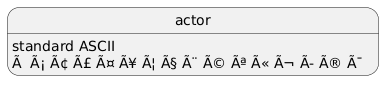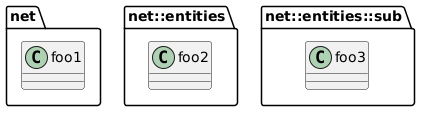# PlantUML tests

### PlantUML site

• Sequential diagram with Cerulean theme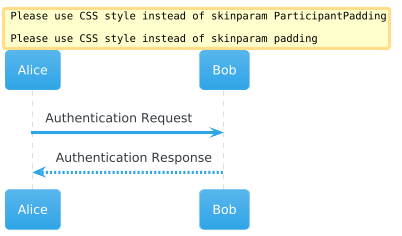• Activity diagram with Cerulean-outline theme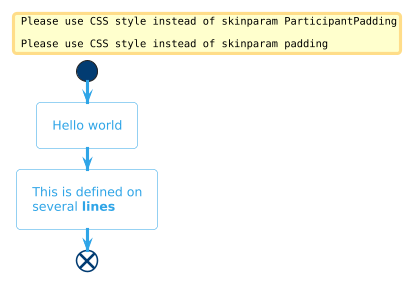• Use Case diagram with Cyborg theme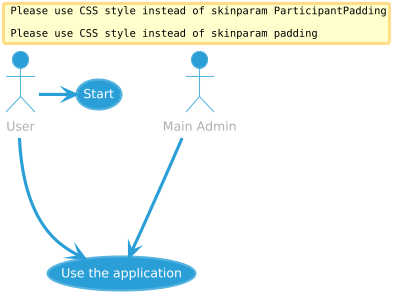• Class diagram with different types of connection and with Materia theme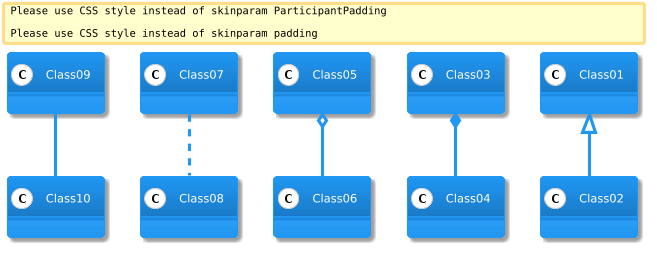• Component diagram with Cyber-outline theme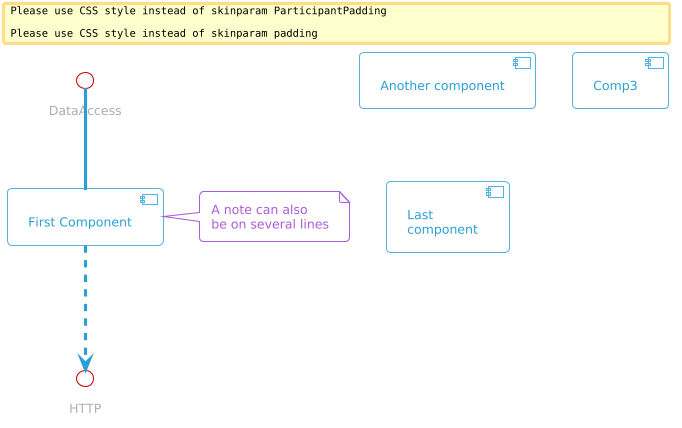• State diagram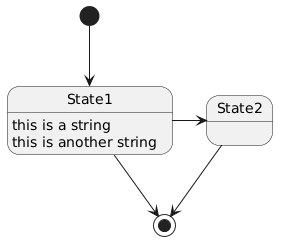• Object diagram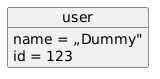• Deployment diagram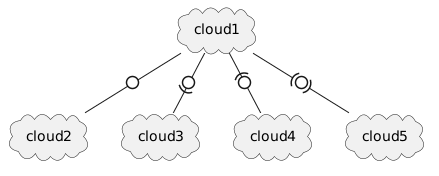• Wireframe diagram• Flowchart diagram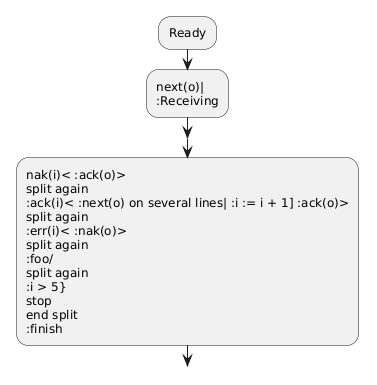• Timing diagram – Why not functioning?• Gantt diagram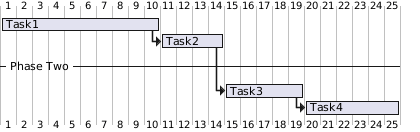• Mindmap diagram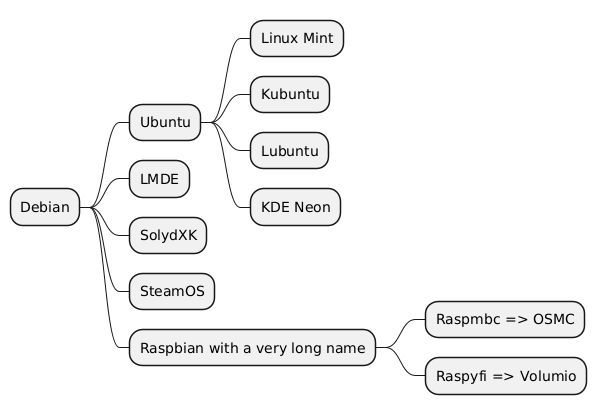• Diataa diagram – the <pre> tag is needed!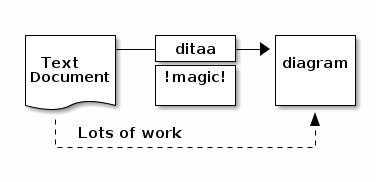• Another Ditaa diagram, another possibility• Another Ditaa diagram, another possibility• Graphviz diagram – dot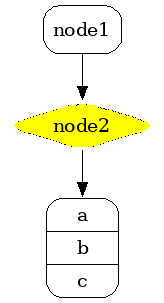• Graphviz diagram – something more complex• WBS diagram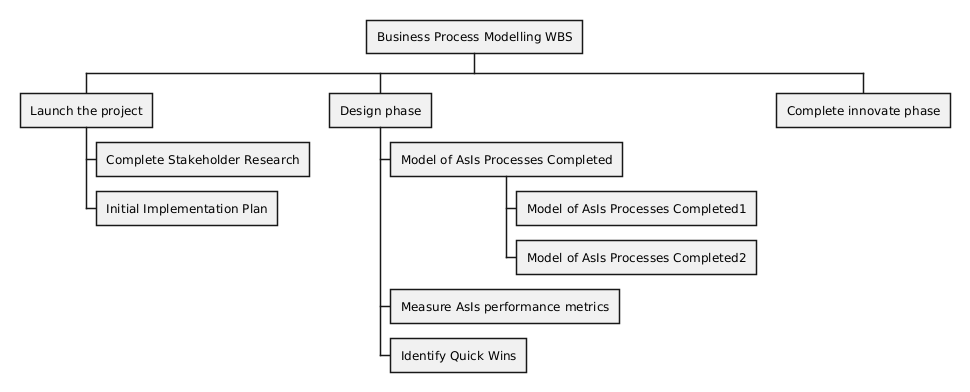• Diagram with included math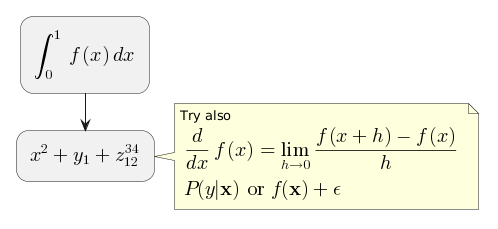• Math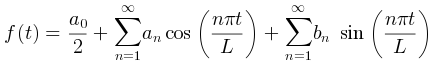• Latex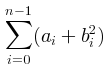• Archimate – not very well 🙁• ER diagram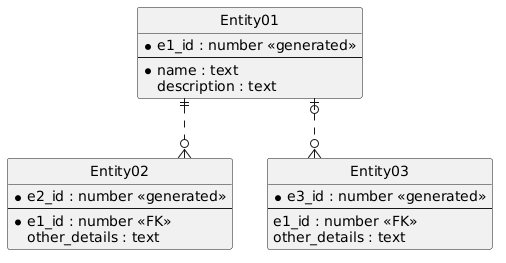• Hand written diagram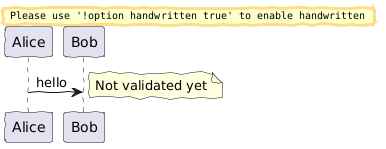• Links can be used in diagrams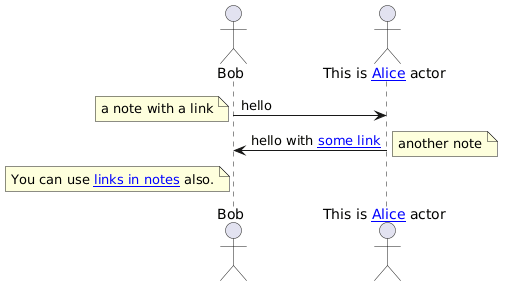• A special command dedicated to Steve Jobs and Steve Wozniak.• Another charset